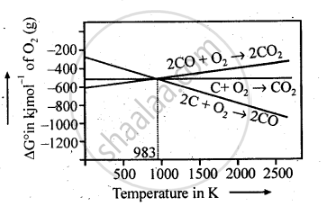Share

# Out of C and CO, Which is a Better Reducing Agent at 673 K? - CBSE (Science) Class 12 - Chemistry

ConceptExtraction of Crude Metal from Concentrated Ore

#### Question

Out of C and CO, which is a better reducing agent at 673 K?

#### Solution 1

At 673 K, the value of triangleG_(CO,CO_2) is less than that of triangleG_(("C,CO")) Therefore, CO can be oxidised more easily to CO2 than C to CO. Hence, CO is a better reducing ag ent than C at 673 K.

#### Solution 2

This can be explained thermodynamically, taking entropy and free energy changes into account.

C(s) + O_2(g) -> CO_2(g)

2C(s) + O_2(g) -> 2CO (g)

Case i: Volume of CO2 produced  = Volume of O2 used.

:.triangleS is very small and triangleG does not change with temperature.

:.Plot of triangleG Vs T is almost hrizontal

Case ii: Volume of CO produced = 2 x Volume of O2 used

:.triangleS is positive and hence triangleG beccomes increasingly negative as the temperature increases.

:.Plot of triangle ""^@G Vs T slopes downwards.As can be seen from ΔG° Vs T plot (Ellingham diagram), lines for the reactions, C ——–> C02 and C ——–> CO cross at 983 K. Below 983 K, the reaction (a) is energetically more favourable but above 673 K, reaction (b) is favourable and preferred. Thus, below 673 K both C and CO can act as a reducing agent but since CO can be more easily oxidised to C02 than C to C02 , therefore, below 673 K, CO is more effective reducing agent than carbon

Is there an error in this question or solution?

#### APPEARS IN

NCERT Solution for Chemistry Textbook for Class 12 (2018 to Current)
Chapter 6: General Principles and Processes of Isolation of Elements
Q: 5 | Page no. 163

#### Video TutorialsVIEW ALL 

Solution Out of C and CO, Which is a Better Reducing Agent at 673 K? Concept: Extraction of Crude Metal from Concentrated Ore.
S